﻿ Fundamentals and Applications of Electrochemical Impedance Spectroscopy - A Didactic PerspectivePublications are Open
Access in this journal
Article Versions
Export Article
• Normal Style
• MLA Style
• APA Style
• Chicago Style
Research Article
Open Access Peer-reviewed

### Fundamentals and Applications of Electrochemical Impedance Spectroscopy - A Didactic Perspective

Achim Habekost
World Journal of Chemical Education. 2021, 9(1), 14-21. DOI: 10.12691/wjce-9-1-3
Received November 19, 2020; Revised December 20, 2020; Accepted December 27, 2020

### Abstract

Two experiments are presented to introduce students to the utility of electrochemical impedance spectroscopy (EIS) in finding electrodes suitable for applications such as battery development. We used different metal and carbon nanotube (CNT) screen-printed electrodes (SPEs) and compared EIS with cyclic voltammetry (CV) curves. In addition, we prepared the SPEs with graphene powder to show the different behaviour of modified and unmodified SPEs with CV and EIS.

### 1. Introduction

Along with other electrochemical measurements such as cyclic voltammetry (CV), square wave voltammetry (SWV) or chronoamperometry (CA), electrochemical impedance spectroscopy (EIS) plays an important role in measuring the characteristics of electrode in electrolyte solutions.

If a potential is applied to an electrode-electrolyte interface, a flow of charge and matter occurs. Without going into details, in EIS the impedance (the resistance of the AC circuit) of the electrochemical system is measured as a function of the applied frequency. The processes at the electrode, immersed in the solution, can be described with different electrical compounds as resistors (solution resistor, Rs; charge transfer or polarization resistor, Rp), capacitor (Helmholtz double layer in front of the electrode, Cdl), and coil (inductance resulting from the current, which induces an electromotive force that opposes a change in current, L). One of the main purposes of EIS is to describe an electrochemical system through a combination of these passive electric compounds. In contrast to the ohmic resistor, capacitors and coils have a frequency-dependent resistance, which is inversely proportional to the frequency of the first and proportional to the frequency of the latter.

Ions in the solution diffuse in the alternating current field. At high frequencies the diffusion is negligible because the ions do not move very far. At lower frequencies, however, the ions diffuse farther. This is described by the Warburg impedance.

The potential is described as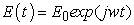and the current response as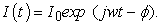(1)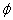: phase between potential and current;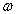: radial frequency = 2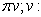frequency, j: imaginary unit.

The impedance, Z, is represented as the complex number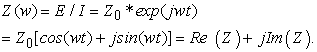(2)

Re: real part and Im: imaginary part of the impedance.

Therefore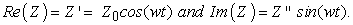(3)

The modulus of Z is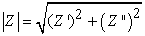(4)

In electrochemistry, a Randles circuit is one of the simplest electrical circuits to describe an electrochemical system. It consists of an electrolyte resistance, RS, in series with the parallel combination of the double-layer capacitance, Cdl, and an impedance of a faradaic (i.e., electron-transfer) reaction, Rp. Sometimes a constant phase element CPE is used instead of an imperfect capacitor, which is in series to Rs or to Rp. The Warburg impedance (ZW) describes the diffusion. In real electrochemical systems, EIS is more complicated and the Randles circuit may not give appropriate results; however, in this article we restrict ourselves on simple, didactical electrochemical systems.

Figure 1 shows the Randles circuit which is often used at the beginning of fitting data.

• Figure 1. Randles circuit [R(RC)W]. Abbreviations: “[...]” means “in series”, “(…)” means “parallel”. Indices are not shown.

Calculating the equivalent circuit without the Warburg impedance is complicated, and leads to 1, 2, 3, 4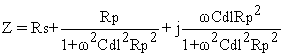(5)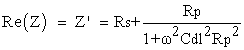and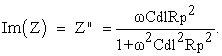The faradaic current i0, caused by a charge-transfer through the electrode interface, is given by eq (6):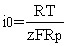(6)

with z: number of electrons implied in the transfer; F: Faraday constant; T: temperature; Rp: charge transfer or polarization resistor.

The charge transfer resistance (Rp) correlates with the charge-transfer rate (k0) and the exchange-current density (i0):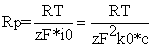(7)

The exchange-current density, i0, is: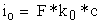(8)

The double layer capacitance can be estimated from Zmax of the semicircle (Figure 2).

When calculating the Warburg impedance (Figure 1, right), it is necessary to distinguish between two cases:

a) Low frequency case: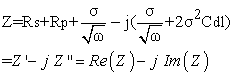And therefore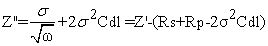(9)

One can see that Z'' is scaled linearly with Z'.

The diffusion coefficient can be calculated from eq (10) 2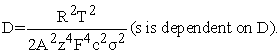(10)

b) High frequency case:

In this case the Warburg impedance equals zero, because the ions do not diffuse. This results in the equation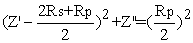(11)

This is a circular equation with the center at Z' = Rs + Rp/2 and radius Rp/2.

In a Nyquist diagram -Im(Z) = -Z'' is plotted against Re(Z) = Z' for different frequencies, from low frequencies on the right side to high frequencies on the left side (Figure 2). Figure 3 shows a three-dimensional plot. Note the shortcoming of the Nyquist plot that the frequency is not displayed.

The maximum of the semicircle is= 101 Hz. Therefore with Rp = 649 Ω Cdl = 1/(2π*101*649) = 2.4 μF, which is a good estimation compared to the fitted value.

Another important presentation is the Bode plot. Here, both the modulus of the impedance, |Z|, and the phase shift on the y-axis are plotted against log frequency on the x-axis. Current through capacitors is phase-shifted by 90 degrees with respect to voltage. In contrast, current through inductors is phase-shifted by -90 degrees with respect to voltage, while the current through a resistor stays in phase with the voltage across the resistor.

Figure 4 shows the Bode plot of the above Randles circuit. One can see that the Bode plot is an superposition of the (RC) circuit and a Warburg impedance (Figure 4, bottom).

• Figure 2. Nyquist diagram. Points: measuring points; solid line: fit with Randles circuit (Rs = 92 Ω, Rp = 649 Ω, Cdl = 2.2 μF, Yo(Warburg) = 3.02 mMho(s)1/2), see insert. Note: “Mho” is Ohm written backwards. Y0 is the admittance (reciprocal of the impedance) multiplied by sqr(ω)
• Figure 3. Three-dimensional plot of Z'' against Z' and frequency
• Figure 4. Top: Bode plot for the same Randles circuit as in Figure 2. Middle: Simulation for RC. Bottom: Simulation for Warburg only

At low frequencies the capacitor blocks (high impedance), while at high frequencies the capacitor has low impedance.

The phase is: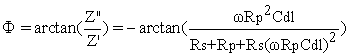(12)

The Warburg impedance scales negative linearily with frequency: At low frequencies the Warburg impedance is high; at high frequences it is low. The phase is constant at 45 degrees.

### 2. Experiments

Chemicals and procedure

K4[Fe(CN)6], 1 mmol/L in 0.1 M aqueous Na2SO4.

Graphene nanoplatelets, purity 91%, particle size up to 2μm: Plasma Chem GmbH PL-P-G750

Instruments: CV and EIS were recorded with a DRP-STAT-I-400 potentiostat with EIS from Metrohm, DropSens. DRP-STAT-I-400 is a dual-channel impedance instrument for multiplexed EIS measurements, controlled by DropView 8400 software. This allows specific EIS features such as data presentation of Nyquist, Bode and Lissajous plots, including information about time domain, frequency domain as well as EIS data treatment, including fit and simulation, semicircle fit, and EIS analysis.

Procedure: A drop of the solution is placed on the SPE with a pipette. The experiments were performed with different SPEs: DS 1110 CNT with two CNT working electrodes and CNT counter electrode, reference electrode: Ag (see Figure 5, right) and Au-AT (working electrode and counter electrode: Au; reference electrode: Ag). The reference potential of the silver electrode is a pseudo-potential.

electrode is a pseudo-potential.

• Figure 5. Left: DS Au-AT (AT means: high temperature curing ink). Right: DS 1110 CNT with two CNT working electrodes

Electrochemical cells are normally non-linear. This means that doubling the potential does not necessarily double the current. In EIS, only small excitation potentials (amplitude) in the range of few 10 mV are used. Therefore, the cell response is (pseudo-)linear. This means that a sinusoidal potential results in a sinusoidal, phase-shifted current.

As mentioned above, different working materials were investigated, including metallic and non-metallic materials. In addition, the electrodes were modified by rubbing graphene on them with a Q Tip.

We compared CV and EIS spectra. Both were fitted: CV with DigiElch (professional)®, and the EIS spectra after defining Randles circuits.

a) Metallic working electrodes with and without graphene

Figure 6 shows the CV of the K4[Fe(CN)6] solution with Au-AT (solid line) and Au-AT decorated with graphene (dotted line).

• Figure 6. CV of K4[Fe(CN)6] with Au-AT (solid line) and with Au-AT / graphene (dotted line).

A comparison of the (fitting) curves shows that the electron transfer is faster when the gold electrode is decorated with graphene (k'0 = 0.0033 cm/s vs k'0 = 0.007 cm/s).

• Figure 7. Left: Au-AT without graphene. Fitting parameters: E0 = 0.14 V; k'0 = 0.0033 cm/s. Right: Au-AT with graphene. Fitting parameters: E0 = 0.17 V; k'0 = 0.007 cm/s. (k0 (eq. (8) and k'0 have different dimensions)

Figure 8 shows the EIS spectra.

• Figure 8. EIS spectra of K4[Fe(CN)6] with Au-AT (pink dots) and with Au-AT / graphene (blue dots). Lines: fitted curves. Insert: Randles circuits

Curve fitting shows that the charge-transfer resistance differs significantly from 62.3 Ω (Au-AT) to 13.1 Ω (Au-AT / graphene). Therefore, the charge-transfer rate (k0 or k'0) is faster with Au-AT / graphene. Theoretical considerations must explain these results, but this goes beyond the scope of this paper.

a) CNT working electrodes with and without graphene

Figure 9 shows the CVs of K4[Fe(CN)6] with CNT both with (dotted line) and without graphene (solid line).

It can clearly be seen that the current peaks rise when the CNT is decorated with graphene. In addition, the current peaks move slightly together. Fitting with DigiElch Professional® confirms this: the rate constant with graphene is higher (k'0 = 0.0046 cm/s vs k'0 = 0.0033 cm/s).

Figure 10 shows the corresponding Nyquist and Bode plots. The Nyquist plot differs between CNT with and without graphene, but the Bode plot, where the modulus of the impedance |Z| is plotted against the frequency, is more meaningful. At each frequency, |Z| is smaller for CNT with graphene than without graphene.

• Figure 9. Top: CV of K4[Fe(CN)6] with CNT-WE without (solid line) and with graphene (dotted line). Bottom: CV and fitted curves. Left: CNT with graphene; k'0 = 0.0046 cm/s. Right: CNT without graphene; k'0 = 0.0033 cm/s
• Figure 10. Top: EIS of K4[Fe(CN)6] with CNT-WE with graphene (blue points) and without graphene (red points). Top: Nyquist diagram. Bottom: Bode plot.

### 3. Conclusion

EIS is a very powerful technique to measure electrochemical behavior and model the results using Randles circuits. The EIS can be easily measured with inexpensive disposable screen-printed electrodes that can be easily modified and compared with CV. Our explorative qualitative study suggests that EIS information together with other electrochemical measurements such as CV and spectroelectrochemistry provide a way to understand electrode processes in a didactic sense.

### Notes

The author declares no competing financial interest.

### Acknowledgments

The author would like to thank the Vector Foundation (Stuttgart, Germany), the Fonds der Chemischen Industrie (Frankfurt, Germany), and the University of Education Ludwigsburg (Germany) for financial support.

### References

  D.D. Macdonald, Reflections on the history of electrochemical impedance spectroscopy, Electrochim. Acta (2006), 51, 1376-1388. In article View Article  E.P. Randviir, C.E. Banks, Electrochemical impedance spectroscopy: an overview of biological applications, J. Anal. Methods (2013), 5, 1098-1115. In article View Article  D. Ende, K-M. Mangold, Impedanzspektroskopie, Chemie in unserer Zeit (1993), 27, 134-140. In article View Article  Andrzej Lasla, Electrochemical Impedance Spectroscopy and its Applications, Springer, New York, 2014. In article View ArticleThis work is licensed under a Creative Commons Attribution 4.0 International License. To view a copy of this license, visit http://creativecommons.org/licenses/by/4.0/